$$\newcommand{\id}{\mathrm{id}}$$ $$\newcommand{\Span}{\mathrm{span}}$$ $$\newcommand{\kernel}{\mathrm{null}\,}$$ $$\newcommand{\range}{\mathrm{range}\,}$$ $$\newcommand{\RealPart}{\mathrm{Re}}$$ $$\newcommand{\ImaginaryPart}{\mathrm{Im}}$$ $$\newcommand{\Argument}{\mathrm{Arg}}$$ $$\newcommand{\norm}{\| #1 \|}$$ $$\newcommand{\inner}{\langle #1, #2 \rangle}$$ $$\newcommand{\Span}{\mathrm{span}}$$

# 5.5: Solve Mixture Applications with Systems of Equations

$$\newcommand{\vecs}{\overset { \rightharpoonup} {\mathbf{#1}} }$$ $$\newcommand{\vecd}{\overset{-\!-\!\rightharpoonup}{\vphantom{a}\smash {#1}}}$$$$\newcommand{\id}{\mathrm{id}}$$ $$\newcommand{\Span}{\mathrm{span}}$$ $$\newcommand{\kernel}{\mathrm{null}\,}$$ $$\newcommand{\range}{\mathrm{range}\,}$$ $$\newcommand{\RealPart}{\mathrm{Re}}$$ $$\newcommand{\ImaginaryPart}{\mathrm{Im}}$$ $$\newcommand{\Argument}{\mathrm{Arg}}$$ $$\newcommand{\norm}{\| #1 \|}$$ $$\newcommand{\inner}{\langle #1, #2 \rangle}$$ $$\newcommand{\Span}{\mathrm{span}}$$ $$\newcommand{\id}{\mathrm{id}}$$ $$\newcommand{\Span}{\mathrm{span}}$$ $$\newcommand{\kernel}{\mathrm{null}\,}$$ $$\newcommand{\range}{\mathrm{range}\,}$$ $$\newcommand{\RealPart}{\mathrm{Re}}$$ $$\newcommand{\ImaginaryPart}{\mathrm{Im}}$$ $$\newcommand{\Argument}{\mathrm{Arg}}$$ $$\newcommand{\norm}{\| #1 \|}$$ $$\newcommand{\inner}{\langle #1, #2 \rangle}$$ $$\newcommand{\Span}{\mathrm{span}}$$

Learning Objectives

By the end of this section, you will be able to:

• Solve mixture applications
• Solve interest applications

Note

Before you get started, take this readiness quiz.

1. Multiply 4.025(1,562).
If you missed this problem, review Exercise 1.8.22.
2. Write 8.2% as a decimal.
If you missed this problem, review Exercise 1.8.46.
3. Earl’s dinner bill came to $32.50 and he wanted to leave an 18% tip. How much should the tip be? If you missed this problem, review Exercise 3.2.10. ## Solve Mixture Applications When we solved mixture applications with coins and tickets earlier, we started by creating a table so we could organize the information. For a coin example with nickels and dimes, the table looked like this:Using one variable meant that we had to relate the number of nickels and the number of dimes. We had to decide if we were going to let n be the number of nickels and then write the number of dimes in terms of n, or if we would let d be the number of dimes and write the number of nickels in terms of d. Now that we know how to solve systems of equations with two variables, we’ll just let n be the number of nickels and d be the number of dimes. We’ll write one equation based on the total value column, like we did before, and the other equation will come from the number column. For the first example, we’ll do a ticket problem where the ticket prices are in whole dollars, so we won’t need to use decimals just yet. Exercise $$\PageIndex{1}$$ Translate to a system of equations and solve: The box office at a movie theater sold 147 tickets for the evening show, and receipts totaled$1,302. How many $11 adult and how many$8 child tickets were sold?

 Step 1. Read the problem. We will create a table to organize the information. Step 2. Identify what we are looking for. We are looking for the number of adult tickets and the number of child tickets sold. Step 3. Name what we are looking for. Let a= the number of adult tickets. c= the number of child tickets A table will help us organize the data. We have two types of tickets: adult and child. Write a and c for the number of tickets. Write the total number of tickets sold at the bottom of the Number column. Altogether 147 were sold. Write the value of each type of ticket in the Value column. The value of each adult ticket is $11. The value of each child tickets is$8. The number times the value gives the total value, so the total value of adult tickets is $$a\cdot 11=11a$$, and the total value of child tickets is $$c\cdot 8=8c$$.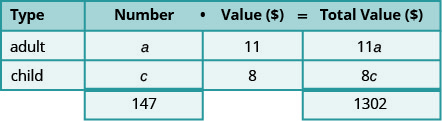Altogether the total value of the tickets was $1,302. Fill in the Total Value column. Step 4. Translate into a system of equations. The Number column and the Total Value column give us the system of equations. We will use the elimination method to solve this system.Multiply the first equation by −8.Simplify and add, then solve for a.Substitute a = 42 into the first equation, then solve for c.Step 5. Check the answer in the problem. 42 adult tickets at$11 per ticket makes $462 105 child tickets at$8 per ticket makes $840. The total receipts are$1,302.✓ Step 6. Answer the question. The movie theater sold 42 adult tickets and 105 child tickets.

Exercise $$\PageIndex{2}$$

Translate to a system of equations and solve:

The ticket office at the zoo sold 553 tickets one day. The receipts totaled $3,936. How many$9 adult tickets and how many $6 child tickets were sold? Answer There were 206 adult tickets sold and 347 children tickets sold. Exercise $$\PageIndex{3}$$ Translate to a system of equations and solve: A science center sold 1,363 tickets on a busy weekend. The receipts totaled$12,146. How many $12 adult tickets and how many$7 child tickets were sold?

There were 521 adult tickets sold and 842 children tickets sold.

In Exercise $$\PageIndex{4}$$ we’ll solve a coin problem. Now that we know how to work with systems of two variables, naming the variables in the ‘number’ column will be easy.

Exercise $$\PageIndex{4}$$

Translate to a system of equations and solve:

Priam has a collection of nickels and quarters, with a total value of $7.30. The number of nickels is six less than three times the number of quarters. How many nickels and how many quarters does he have? Answer  Step 1. Read the problem. We will create a table to organize the information. Step 2. Identify what we are looking for. We are looking for the number of nickels and the number of quarters. Step 3. Name what we are looking for. Let n= the number of nickels. q= the number of quarters A table will help us organize the data. We have two types of coins, nickels and quarters. Write n and q for the number of each type of coin. Fill in the Value column with the value of each type of coin. The value of each nickel is$0.05. The value of each quarter is $0.25. The number times the value gives the total value, so, the total value of the nickels is n (0.05) = 0.05n and the total value of quarters is q(0.25) = 0.25q. Altogether the total value of the coins is$7.30.Step 4. Translate into a system of equations. The Total value column gives one equation.We also know the number of nickels is six less than three times the number of quarters. Translate to get the second equation.Now we have the system to solve.Step 5. Solve the system of equations We will use the substitution method. Substitute n = 3q − 6 into the first equation. Simplify and solve for q.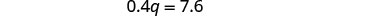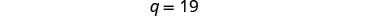To find the number of nickels, substitute q = 19 into the second equation.Step 6. Check the answer in the problem. \begin{aligned} 19 \text { quarters at } \ 0.25 &=\ 4.75 \\ 51 \text { ickels at } \ 0.05 &=\ 2.55 \\ \text { Total } &=\ 7.30 \checkmark \\ 3 \cdot 19-16 &=51 \checkmark\end{aligned} Step 7. Answer the question. Priam has 19 quarters and 51 nickels.

Exercise $$\PageIndex{5}$$

Translate to a system of equations and solve:

Matilda has a handful of quarters and dimes, with a total value of $8.55. The number of quarters is 3 more than twice the number of dimes. How many dimes and how many quarters does she have? Answer Matilda has 13 dimes and 29 quarters. Exercise $$\PageIndex{6}$$ Translate to a system of equations and solve: Juan has a pocketful of nickels and dimes. The total value of the coins is$8.10. The number of dimes is 9 less than twice the number of nickels. How many nickels and how many dimes does Juan have?

Juan has 36 nickels and 63 dimes.

Some mixture applications involve combining foods or drinks. Example situations might include combining raisins and nuts to make a trail mix or using two types of coffee beans to make a blend.

Exercise $$\PageIndex{7}$$

Translate to a system of equations and solve:

Carson wants to make 20 pounds of trail mix using nuts and chocolate chips. His budget requires that the trail mix costs him $7.60 per pound. Nuts cost$9.00 per pound and chocolate chips cost 2.00 per pound. How many pounds of nuts and how many pounds of chocolate chips should he use? Answer  Step 1. Read the problem. We will create a table to organize the information. Step 2. Identify what we are looking for. We are looking for the number of pounds of nuts and the number of pounds of chocolate chips. Step 3. Name what we are looking for. Let n= the number of pound of nuts. c= the number of pounds of chips Carson will mix nuts and chocolate chips to get trail mix. Write in n and c for the number of pounds of nuts and chocolate chips. There will be 20 pounds of trail mix. Put the price per pound of each item in the Value column. Fill in the last column usingNumber · Value = Total Value Step 4. Translate into a system of equations. We get the equations from the Number and Total Value columns.Step 5. Solve the system of equations We will use elimination to solve the system. Multiply the first equation by −2 to eliminate c.Simplify and add. Solve for n.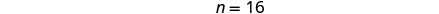To find the number of pounds of chocolate chips, substitute n = 16 into the first equation, then solve for c.c=4 Step 6. Check the answer in the problem. \begin{aligned} 16+4 &=20 \checkmark \\ 9 \cdot 16+2 \cdot 4 &=152 \checkmark \end{aligned} Step 7. Answer the question. Carson should mix 16 pounds of nuts with 4 pounds of chocolate chips to create the trail mix. Exercise $$\PageIndex{8}$$ Translate to a system of equations and solve: Greta wants to make 5 pounds of a nut mix using peanuts and cashews. Her budget requires the mixture to cost her6 per pound. Peanuts are $4 per pound and cashews are$9 per pound. How many pounds of peanuts and how many pounds of cashews should she use?

Greta should use 3 pounds of peanuts and 2 pounds of cashews.

Exercise $$\PageIndex{9}$$

Translate to a system of equations and solve:

Sammy has most of the ingredients he needs to make a large batch of chili. The only items he lacks are beans and ground beef. He needs a total of 20 pounds combined of beans and ground beef and has a budget of $3 per pound. The price of beans is$1 per pound and the price of ground beef is $5 per pound. How many pounds of beans and how many pounds of ground beef should he purchase? Answer Sammy should purchase 10 pounds of beans and 10 pounds of ground beef. Another application of mixture problems relates to concentrated cleaning supplies, other chemicals, and mixed drinks. The concentration is given as a percent. For example, a 20% concentrated household cleanser means that 20% of the total amount is cleanser, and the rest is water. To make 35 ounces of a 20% concentration, you mix 7 ounces (20% of 35) of the cleanser with 28 ounces of water. For these kinds of mixture problems, we’ll use percent instead of value for one of the columns in our table. Exercise $$\PageIndex{10}$$ Translate to a system of equations and solve: Sasheena is a lab assistant at her community college. She needs to make 200 milliliters of a 40% solution of sulfuric acid for a lab experiment. The lab has only 25% and 50% solutions in the storeroom. How much should she mix of the 25% and the 50% solutions to make the 40% solution? Answer  Step 1. Read the problem. A figure may help us visualize the situation, then we will create a table to organize the information. Sasheena must mix some of the 25% solution and some of the 50% solution together to get 200 ml of the 40% solution.Step 2. Identify what we are looking for. We are looking for how much of each solution she needs. Step 3. Name what we are looking for. Let x= number of ml of 25% solution. y= number of ml of 50% solution A table will help us organize the data. She will mix x ml of 25% with y ml of 50% to get 200 ml of 40% solution. We write the percents as decimals in the chart. We multiply the number of units times the concentration to get the total amount of sulfuric acid in each solution.Step 4. Translate into a system of equations. We get the equations from the Number column and the Amount column. Now we have the system.Step 5. Solve the system of equations. We will solve the system by elimination. Multiply the first equation by −0.5 to eliminate y.Simplify and add to solve for x.To solve for y, substitute x = 80 into the first equation.Step 6. Check the answer in the problem. $$\begin{array}{rll} 80+120 &=&120 \checkmark\\ 0.25(80)+0.50(120) &=&80 \checkmark \\ &&\text{Yes!} \end{array}$$ Step 7. Answer the question. Sasheena should mix 80 ml of the 25% solution with 120 ml of the 50% solution to get the 200 ml of the 40% solution. Exercise $$\PageIndex{11}$$ Translate to a system of equations and solve: LeBron needs 150 milliliters of a 30% solution of sulfuric acid for a lab experiment but only has access to a 25% and a 50% solution. How much of the 25% and how much of the 50% solution should he mix to make the 30% solution? Answer LeBron needs 120 ml of the 25% solution and 30 ml of the 50% solution. Exercise $$\PageIndex{12}$$ Translate to a system of equations and solve: Anatole needs to make 250 milliliters of a 25% solution of hydrochloric acid for a lab experiment. The lab only has a 10% solution and a 40% solution in the storeroom. How much of the 10% and how much of the 40% solutions should he mix to make the 25% solution? Answer Anatole should mix 125 ml of the 10% solution and 125 ml of the 40% solution. ## Solve Interest Applications The formula to model interest applications is I = Prt. Interest, I, is the product of the principal, P, the rate, r, and the time, t. In our work here, we will calculate the interest earned in one year, so t will be 1. We modify the column titles in the mixture table to show the formula for interest, as you’ll see in Exercise $$\PageIndex{13}$$. Exercise $$\PageIndex{13}$$ Translate to a system of equations and solve: Adnan has$40,000 to invest and hopes to earn 7.1% interest per year. He will put some of the money into a stock fund that earns 8% per year and the rest into bonds that earns 3% per year. How much money should he put into each fund?

 Step 1. Read the problem. A chart will help us organize the information. Step 2. Identify what we are looking for. We are looking for the amount to invest in each fund. Step 3. Name what we are looking for. Let s= the amount invested in stocks. b= the amount invested in bonds. Write the interest rate as a decimal for each fund. Multiply: Principal · Rate · Time to get the Interest.Step 4. Translate into a system of equations. We get our system of equations from the Principal column and the Interest column.Step 5. Solve the system of equations Solve by elimination. Multiply the top equation by −0.03.Simplify and add to solve for s.To find b, substitute s = 32,800 into the first equation.Step 6. Check the answer in the problem. We leave the check to you. Step 7. Answer the question. Adnan should invest $32,800 in stock and$7,200 in bonds.
Did you notice that the Principal column represents the total amount of money invested while the Interest column represents only the interest earned? Likewise, the first equation in our system, s + b = 40,000, represents the total amount of money invested and the second equation, 0.08s + 0.03b = 0.071(40,000), represents the interest earned.

Exercise $$\PageIndex{14}$$

Translate to a system of equations and solve:

Leon had $50,000 to invest and hopes to earn 6.2 % interest per year. He will put some of the money into a stock fund that earns 7% per year and the rest in to a savings account that earns 2% per year. How much money should he put into each fund? Answer Leon should put$42,000 in the stock fund and $8000 in the savings account. Exercise $$\PageIndex{15}$$ Translate to a system of equations and solve: Julius invested$7,000 into two stock investments. One stock paid 11% interest and the other stock paid 13% interest. He earned 12.5% interest on the total investment. How much money did he put in each stock?

Julius invested $1,750 at 11% and$5,250 at 13%.

Exercise $$\PageIndex{16}$$

Translate to a system of equations and solve:

Rosie owes $21,540 on her two student loans. The interest rate on her bank loan is 10.5% and the interest rate on the federal loan is 5.9%. The total amount of interest she paid last year was$1,669.68. What was the principal for each loan?

 Step 1. Read the problem. A chart will help us organize the information. Step 2. Identify what we are looking for. We are looking for the principal of each loan. Step 3. Name what we are looking for. Let b= the principal for the bank loan. f= the principal on the federal loan The total loans are $21,540. Record the interest rates as decimals in the chart.Multiply using the formula l = Pr t to get the Interest. Step 4. Translate into a system of equations. The system of equations comes from the Principal column and the Interest column.Step 5. Solve the system of equations We will use substitution to solve. Solve the first equation for b.Substitute b = −f + 21,540 into the second equation.Simplify and solve for f.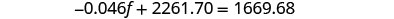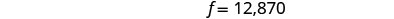To find b, substitute f = 12,870 into the first equation.Step 6. Check the answer in the problem. We leave the check to you. Step 7. Answer the question. The principal of the bank loan is$12,870 and the principal for the federal loan is $8,670. Exercise $$\PageIndex{17}$$ Translate to a system of equations and solve: Laura owes$18,000 on her student loans. The interest rate on the bank loan is 2.5% and the interest rate on the federal loan is 6.9 %. The total amount of interest she paid last year was $1,066. What was the principal for each loan? Answer The principal amount for the bank loan was$4,000. The principal amount for the federal loan was $14,000. Exercise $$\PageIndex{18}$$ Translate to a system of equations and solve: Jill’s Sandwich Shoppe owes$65,200 on two business loans, one at 4.5% interest and the other at 7.2% interest. The total amount of interest owed last year was $3,582. What was the principal for each loan? Answer The principal amount for was$41,200 at 4.5%. The principal amount was, \$24,000 at 7.2%.

Note

Access these online resources for additional instruction and practice with solving application problems with systems of linear equations.

## Key Concepts

• Table for coin and mixture applications• Table for concentration applications• Table for interest applications